Home  /  Products  /  Stata 12  /  Multivariate GARCH

## Multivariate GARCH

MGARCH stands for multivariate GARCH, or multivariate generalized autoregressive conditional heteroskedasticity. MGARCH allows the conditional-on-past-history covariance matrix of the dependent variables to follow a flexible dynamic structure.

Stata’s new mgarch command fits MGARCH models. mgarch implements diagonal vech and conditional correlation models. Conditional correlation models are also new to Stata 12. Conditional correlation models use nonlinear combinations of univariate GARCH models to represent the conditional covariances. mgarch provides estimators for three popular conditional correlation models—CCC, DCC, VCC—also known as constant, dynamic, and varying conditional correlation.

Below we analyze daily data on returns of Toyota, Nissan, and Honda stocks. We include the lag of the Nissan stock in the mean equation for Honda. We specify one ARCH term and one GARCH term for the conditional variance equation of each company.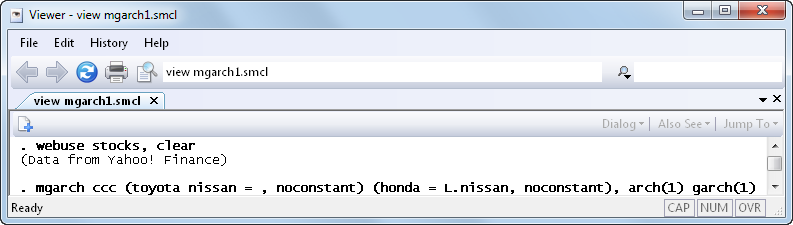And the results are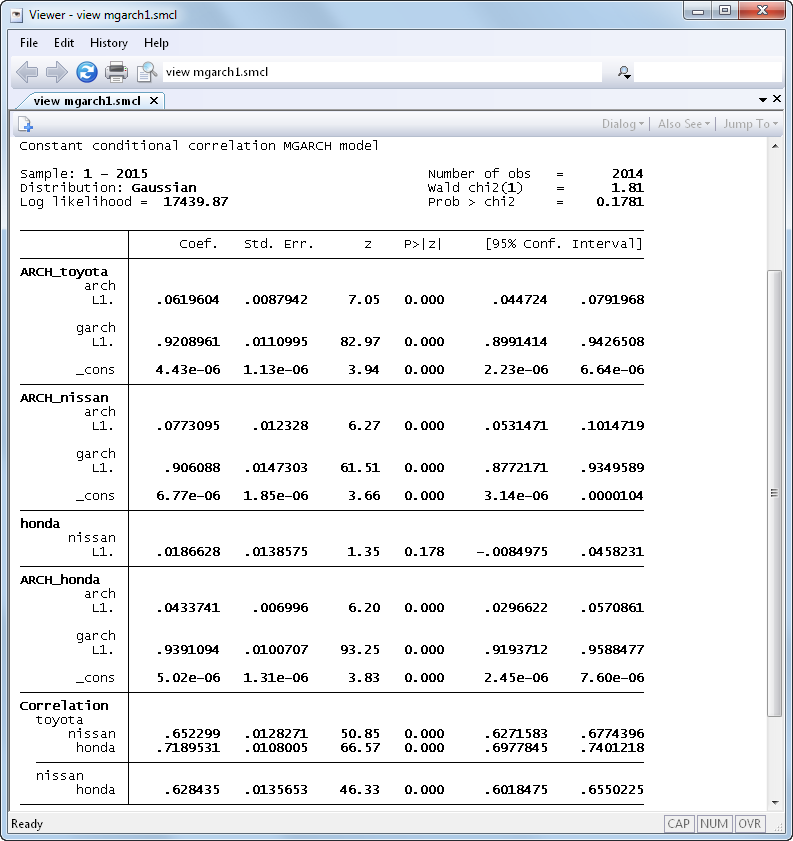Having estimated our model, we can now forecast the conditional variances 50 time periods into the future.

. tsappend, add(50)
. predict H*, variance dynamic(2016)


We can graph the result: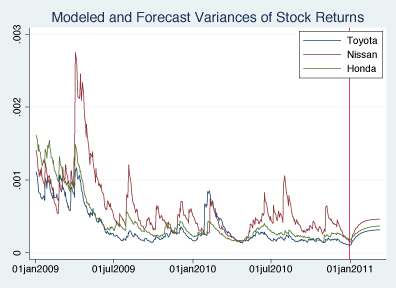Above, we fit a CCC model. We could instead fit a DCC model in which the correlation matrix at each time period is modeled as a weighted average of its own past and recent shocks.

Below we fit a bivariate model of stock returns and specify that the error term follows a multivariate Student’s t distribution: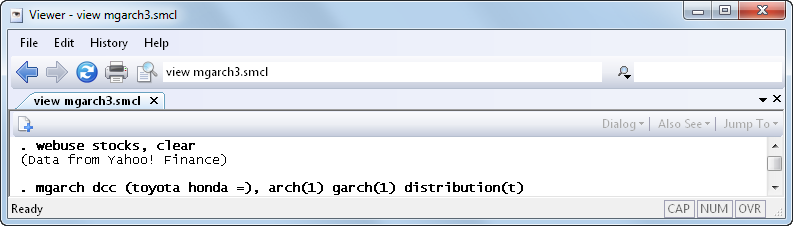And the results are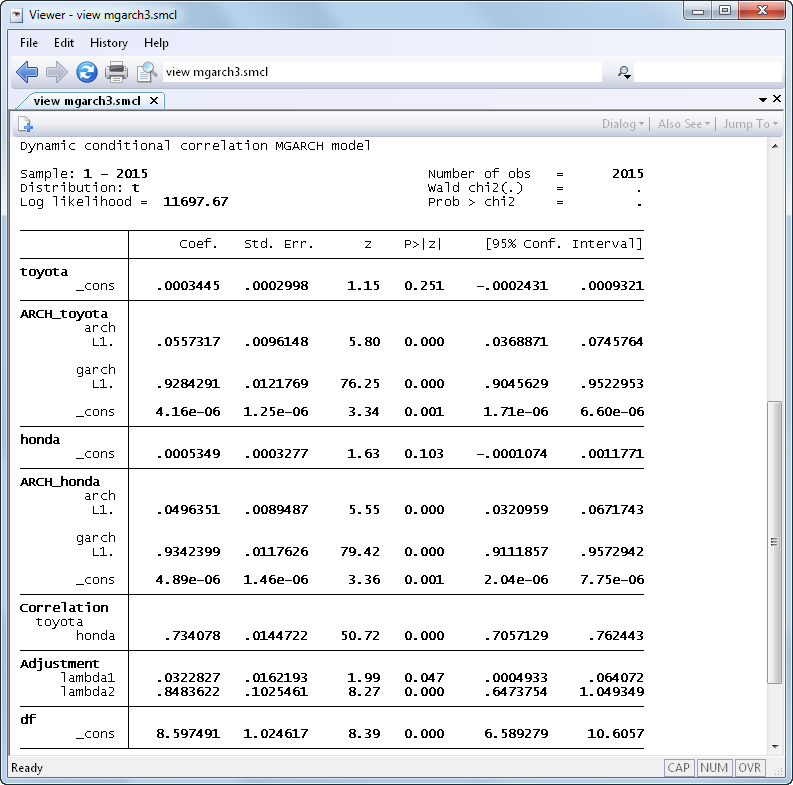The DCC model reduces to the CCC model when the adjustment parameters that govern the dynamic correlation process are jointly equal to zero. We can perform a Wald test to test this hypothesis.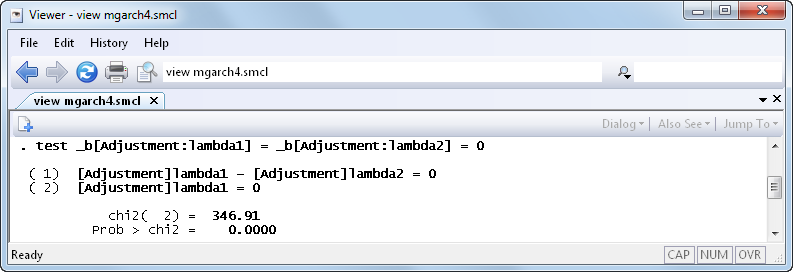We have fit a CCC model and a DCC model. We could fit a VCC model in which the correlation matrix is modeled as a weighted average of its own past and averages of recent shocks.

To illustrate the flexibility of the conditional correlation estimators, we specify each variance equation separately in the mgarch command below. We include two ARCH terms, one GARCH term, and an independent variable in the variance equation of Honda and one ARCH term for the variance equation of Toyota.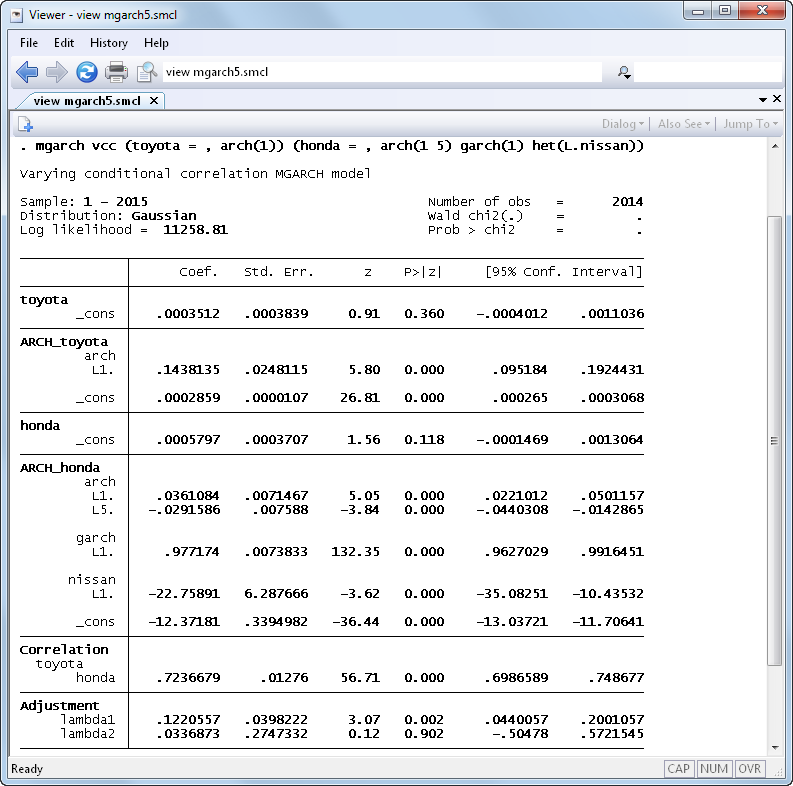We have demonstrated CCC, DCC, and VCC. We could also demonstrate diagonal vech, except Stata 11 could do that. New in Stata 12 are multivariate Student’s t errors in the diagonal vech model.

For a complete list of what’s new in time-series analysis, click here.

See New in Stata 18 to learn about what was added in Stata 18.